# Eigenvalues of the p,q,r-Laplacian with a parametric boundary condition

### Full PDFcarpathian_2022_38_3_547_561

Issue no:

Consider in a bounded domain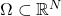,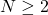, with smooth boundary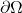the following nonlinear eigenvalue problem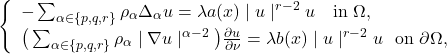where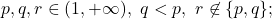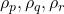are positive constants;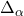is the usual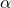-Laplacian, i.e.,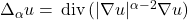;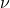is the unit outward normal to;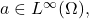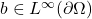{are given nonnegative functions satisfying}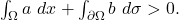Such a triple-phase problem is motivated by some models arising in mathematical physics.

If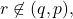we determine a positive number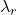such that the set of eigenvalues of the above problem is precisely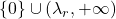. On the other hand, in the complementary case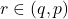with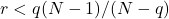if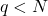, we prove that
there exist two positive constants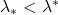such that any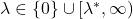is an eigenvalue of the above problem, while the set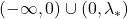contains no eigenvalue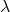of the problem.